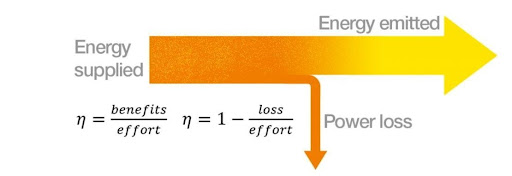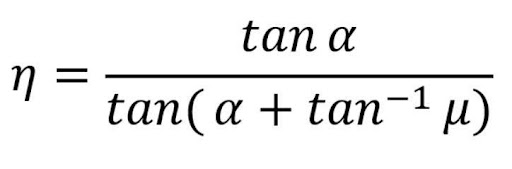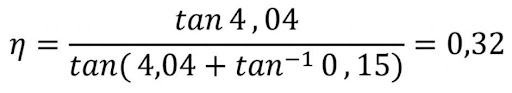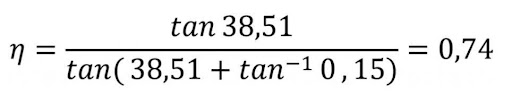# How to calculate efficiency of leadscrew??

#### Shery George | 19 October 2021In general, efficiency indicates how high the effectiveness of a device is when conversion losses are taken into account. The efficiency describes the relation between the delivered power (effective output) and the supplied power or loss and expenditure. In the calculation, the efficiency is abbreviated with a small eta (η). The result of the efficiency depends on what is considered a benefit or loss and what is considered an expenditure.

The efficiency shall have a value between 0 and < 1 or, expressed as a percentage, between 0 and < 100%. The efficiency is influenced by various factors. For example, energy is lost through frictional heat, so the effective output is less than the supplied power. The higher the efficiency, the better the supplied energy is transformed into effective output.

How does the efficiency rate of igus® plastic lead screw nuts?

Our tribologically optimised polymer lead screw nuts are characterised by low friction values and higher efficiencies compared to lead screw nuts made of standard plastics such as POM and unlubricated bronze lead screw nuts.

For lead screws, the following formula can be used to calculate the efficiency:η (eta) = efficiency [-]

α (alpha) = angle thread pitch [°]

µ (mü) = coefficient of friction [-]

The efficiency thus depends on the angle of the thread pitch and the coefficient of friction. The trapezoidal thread TR18x4 and a lead screw nut made of iglidur® J illustrate this very well:

α= tan-1 (P/U)

P=pitch= 4mm

U=circumference=π x d= 56.52mm

α= tan-1 (4/56.52) = 4.04°

µ = 0.15 (iglidur® J, dry operation)η = 0.32 or efficiency 32%

Single start trapezoidal lead screw nuts achieve efficiencies between 20% and 48% in dry operation. Ball screw drives achieve efficiencies of up to over 90%, depending on the system.

dryspin® high helix lead screw nuts achieve efficiencies between 35% and 80% in dry operation:

α= tan-1 (P/U)

P=pitch= 25mm

U=circumference=π x d= 31.42mm

α= tan-1 (25/31.42) = 38.51°

µ = 0.15 (iglidur® J, dry operation)

η = 0.74 or efficiency 74%If you want to know more about dryspin leadscrew please go through below link & also you can post your query on arai@igus.net else you can comment on my post.